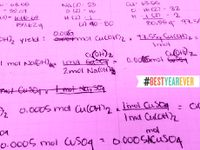George Lucas Educational Foundation
Professional Learning

# How to Creatively Integrate Science and Math

Making a case for more collaboration between math and science teachers.
November 11, 2011Why is the sky blue? I remember in my physical science class, our teacher showed us a possible reason why the sky is blue. He took a canister of liquid oxygen and poured it out on the table. I saw the blueness of the liquid as it flowed out and then disappeared. Then we talked about color, frequencies, and absorption, reflected and radiated light. I wondered how scientist ever figured these things out? Duh -- math! How can you really teach science without math? It is impossible. Science is the application of math.

In science, geometric principles such as symmetry, reflection, shape, and structure reach down to the atomic levels. In science, algebraic balance is required in chemical formulas, growth ratios, and genetic matrices. In science, math is used to analyze nature, discover its secrets and explain its existence and this is the big problem. Science is so complex and getting more so each day. In order to In math class one of the biggest needs is relevance. Students want to know how they are going to benefit from being able to do calculations. Why not use science to teach math? Since one of the biggest uses of mathematics in science is data gathering and analysis, that is the best place to start. When a teacher gives students a real science problem to solve -- one that requires math tools -- the teacher is giving the students a reason to use math. Math then becomes something useful, not something to be dreaded.

Being able to teach math better and being able to teach science better are powerful reasons for the math and science teacher collaborate with each other. According to a case study conducted by Jennifer Dennis and Mary John O'Hair, another reason that math and science teachers should collaborate is that science helps provide relevance to math that is all too often abstract and isolated calculation operations. Instead of using the word problems out of the book that many dread, why not walk down the hall and talk to the science teacher to find out what math they are using in science class? Ultimately, as another study reported, the students' increased conceptual understanding of math and science is the greatest benefit of math and science teacher collaboration. Conceptual understanding means the students know the bigger picture of why things work in math and science, not just how to make them work.

Unfortunately, knowing that increased teacher collaboration in math and science will benefit students and teachers is not enough. Teachers are so busy that finding time to collaborate is difficult. Add to this, the structure of the school inhibits collaboration when math and science teachers are spread out in a large campus. How do you overcome this? Well, a simple request to the principal might do the trick. Let the principal know what you want to do and show him the evidence and if he can, he will help. Another solution is that even though geographically speaking the math and science teachers may be isolated, everyone has a cellphone. Just call them. Texting, Facebook, Google+, Skype, Google Hangouts, or even email can bring science and math teachers together. There are many options -- just do it!

What are ways you work with your companion subject teacher (math or science) to help students understand math and science better?